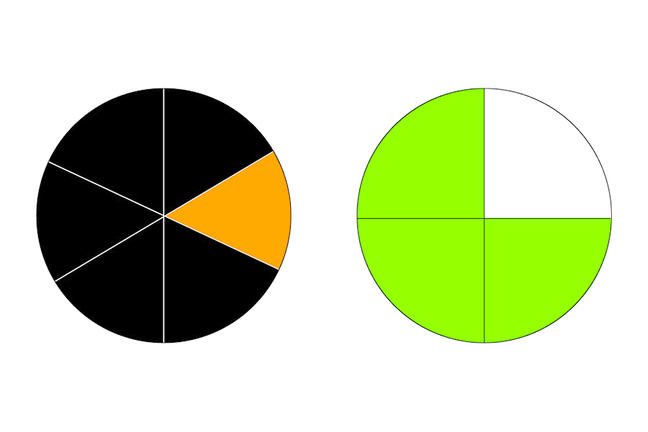## Want to keep learning?

This content is taken from the Cambridge University Press's online course, Teaching Probability. Join the course to learn more.
2.6

## Cambridge University PressThe colours on the spinners represent the outcomes of a trial

# Physical models for frequency

Conditional probability problems like ‘The dog ate my homework’ can involve a complex series of choices and outcomes. To help us model the problem with an experiment, we have chosen specific (but arbitrary) colours to represent events as follows:

• Black to mean that a student is telling the truth
• Orange to mean that a student is lying
• Green to mean that Mr D believes the student’s claim
• White to mean that Mr D accuses the student of lying

These colours are used in two ways in the experiment.

First, they are used to determine the outcome of a trial.

• The spinners shown in the picture above use these colours to decide what happens to each of the students presenting homework excuses.
• We use the first spinner to determine whether the student is telling the truth.
• If the student is lying (Orange on the spinner), Mr D will accuse them of lying.
• If the student is telling the truth (Black on the spinner), we use the second spinner to determine whether Mr D will (wrongly) accuse them of lying.

The colours are also used as a means of recording the results, using interlocking plastic cubes.

• After the first spin, we take a cube of the appropriate colour (black or orange) from a prepared stock.
• If the first spinner was orange (meaning that the student was lying), we join a white cube to the orange cube, to represent the outcome ‘Student lied, was accused’.
• If the first spinner was black, we start with a black cube and join on either a green or white one, depending on the result of the second spinner.
• At the end of the experiment we will have a collection of paired cubes, where the colour combination of each pair represents the ’story’ of an individual student.

—-

Make sure that you understand how this arrangement works (and notice that the probabilities are not the same as in the example that you worked on in the previous step). Do you think that this approach will help students to see what is going on in the experiment?